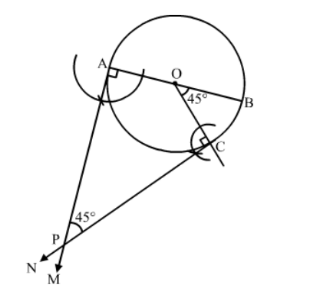# Draw a circle of radius 4.2 cm.`
Question:

Draw a circle of radius 4.2 cm. Draw a pair of tangents to this circle inclined to each other at an angle of 45°.

Solution:

Steps of Construction:

Step 1. Draw a circle with centre O and radius = 4.2 cm.
Step 2. Draw any diameter AOB of this circle.

Step 3. Construct $\angle B O C=45^{\circ}$, such that the radius $O C$ meets the circle at $C$.

Step 4. Draw AM $\perp A B$ and $C N \perp O C$.

AM and CN intersect at P.Thus, PA and PC are the required tangents to the given circle inclined at an angle of 45o.